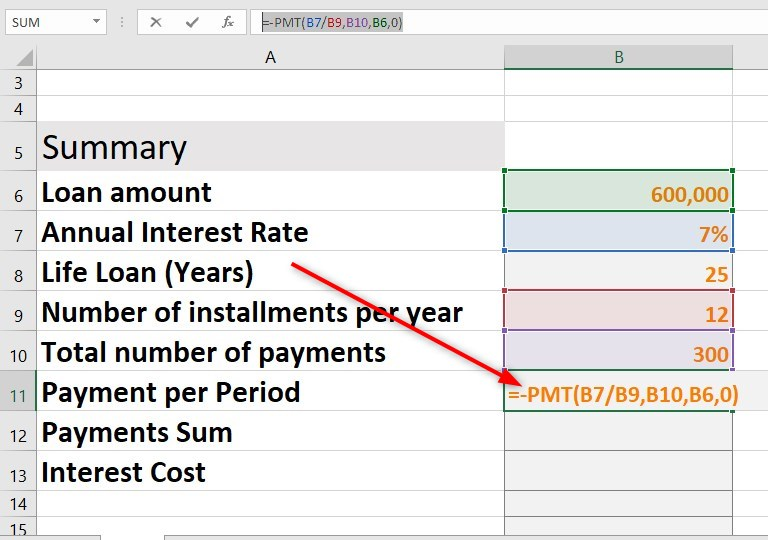# How to Calculate Mortgage Payments in Excel

Excel is a  very useful tool for both personal and business finances. You can use it to calculate mortgage payments, among other day-to-day calculations. Regardless of your Excel skill level, creating a mortgage payment calculator is an easy task.

In this article, I will guide you on calculating mortgage payments in Excel.

## How to create a mortgage calculator

You can create a mortgage calculator by following these steps.

1. Open the Excel Program

2. Click on Blank Document to open a new workbook

3. Write the following categories in column A. But first, adjust the length by dragging the first column to the right of at least 3 cells.

• Loan Amount
• Annual Interest Rate (AIR)
• Life Loan (years)
• Number of installments per year.
• Total Number of Payments
• Payment per Period
• Payments Sum
• Interest Cost

• Loan amount refers to the amount you owe
• The Annual Interest rate refers to the percent interest that accumulates yearly.
• Life loan refers to the total number of years given to repay the loa.n
• Number of installments per year is the total number of times you make payments in one year
• The total number of payments refers to the number of installments per year multiplied by the life loan (years)
• Payment per period stands for a single payment to make every period
• Payment sum refers to the total cost of the loan
• Interest cost stands for the total cost of interest that will accrue in the Life Loan value.

As you can see from the image above, to calculate the total number of payments, I have multiplied life loan years (B8) by the number of installments per year (B9). Therefore, the total number of payments will be 300, as indicated by the image below.

.

5. The next step is to calculate payment per period (Mortgage Monthly Payments)

To achieve this, you need to take advantage of the built-in Excel function called PMT.

The syntax for PMT function is “=PMT(Interest Rate/Payments per Year,Total Number of Payments,Loan Amount,0)”

The formula to use in this case is

=PMT(B7/B9,B10,B6,0)

Press enter to get the results

6. Put a minus sign in front of the PMT to indicate this is the amount to be deducted every month

Therefore, the new formula will be

=-PMT(B7/B9,B10,B6,0)7. Press enter to get the results

8. Next, you need to calculate the total cost of the loan. This refers to the total number of payments multiplied by payment per month/period.

For example, if you make 300 payments of \$4,240.68, then the total cost of the loan will be \$1,272,202.56.

9. Lastly, you need to calculate the Interest Cost. This is straightforward as you need to subtract the loan amount from the sum of payments

For example, in this tutorial, the interest cost is =B12-B6

The interest cost is \$672,202.56

## Mortgage Payment Calculator

Download the Excel file below if you are looking for a ready-to-use Excel Mortgage calculator. All you need is to replace the input sections with your data to get the monthly payments.

## How to calculate Monthly Mortgage Payments in Excel after Down Payment

In the above example, the calculations assume that you have not placed a deposit or downpayment for the house. Therefore, if deposit is required in your case, then the method may not be right for you.

Most financial institutions require someone to place a small down payment, such as 20%, the pay the rest periodically, e.g., per month for certain years. The formula that you will need for this is the PMT function.

Here are the steps to follow.

1. Let’s use the following data to calculate mortgage payment

2. To get the down payment, multiply C4 by C7. C4 contains the loan cost, while C7 contains the down payment in percentage.

=C4*C7

3. To calculate the loan amount, subtract the deposit(Down Payment) from the cost. =C4-C9

4. Lastly, to get the monthly payment, you need to use the following formula

### Mortgage Payment Calculation Formula

=PMT(rate/12,term*12,-C9)

Explanation

PMT FunctionThe PMT function in Excel calculates the periodic payment required to repay a loan over a specified period of time. The syntax for the PMT function is:

PMT(rate, nper, pv, fv, type)

Where:

• rate is the interest rate per period as a decimal.
• nper is the number of payments.
• pv is the present value of the loan.
• fv is the future value of the loan, which is 0 if you want to pay off the loan in full.
• type is a number that specifies when payments are due. 0 means payments are due at the end of each period, and 1 means payments are due at the beginning of each period.

For example, if you borrow \$10,000 at 10% interest for 5 years, the monthly payment would be \$206.20.

5. For this tutorial, we will use the following custom formula

=PMT(rate/12,term*12,-C9)

=PMT(C5/12,C6*12,-C9)

6. As you can see from the image above, the monthly payment will be \$2,338.36